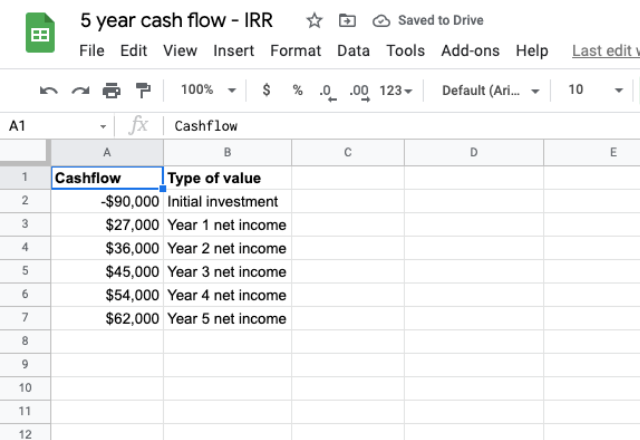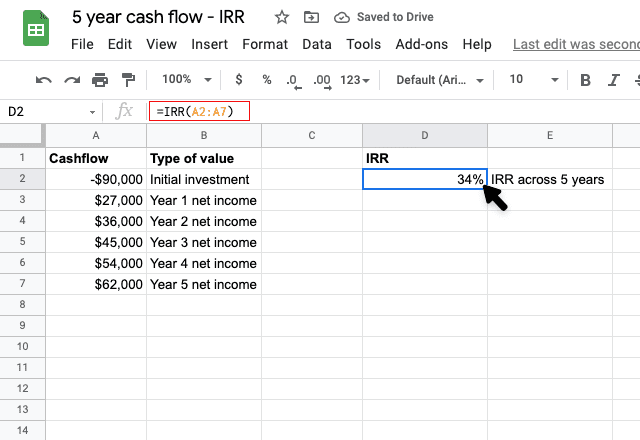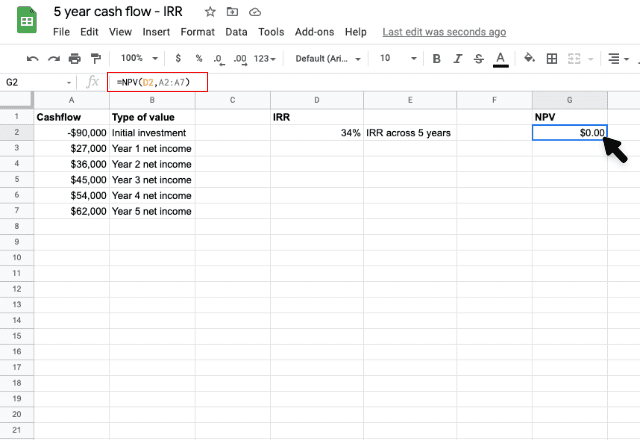# How to use the IRR formula in Google SheetsWritten by
Holly MeikleIf you’re managing the returns of your periodic cash flows in Google Sheets, then using the IRR formula can not only help to streamline your calculations process but also guarantee accurate results.

The IRR formula is usually used in a financial modeling or financial investment context. For example, you can forecast and evaluate your business performance by calculating the return rate based on your initial investment and previous net income. On the other hand, the IRR formula can also help you choose what to invest in; a higher IRR rate equals a better investment opportunity.

In this article, we’ll explore what the IRR formula is, how it works, and how you can employ it in your own cash flow data.

## What is the IRR formula?

The IRR formula stands for ‘Internal Rate of Return’. This formula calculates the interest rate received for an investment consisting of both outgoing payments and income payments that occur at regular periods. It doesn’t consider the external factors, such as inflation or the cost of capital. Hence the term ‘internal’.

Therefore, you can use the IRR formula with the following:

• Periodic payments: regular payments that come in, such as monthly or annually. If you’re looking to calculate return on investment with non-periodic cash flows, use the IXRR formula.
• Uneven cash flow: you don’t have to have even values at every interval for the IRR formula to work

### Syntax

The IRR formula uses the following syntax to calculate your return:

=IRR(cashflow_values, [rate_guess])
• Cashflow_values = the range of cells that contain the outgoing and incoming payments relating to your return of investment

The range must contain at least one positive value (income) and one negative value (payment).
Make sure the values are in the correct order of cash flow
Your range must include numbers only. Any other values, such as text or empty cells, will be ignored.

• [rate_guess] = optional. Estimate of the internal rate of return.

For the majority of instances, you don’t need to provide a guess value for the IRR calculation. If so, the default value will be 0.1 (10%).
You may receive a #NUM! error value if the [rate_guess] doesn’t work. There are 2 reasons this may occur:

1. You have not entered at least one negative and one positive value.
2. The formula couldn’t find an accurate enough result. IRR cycles through the calculation until the result is accurate within 0.00001%. If the formula cannot find a result that works after 20 tries, the #NUM! value will show.

If the result isn’t close to what you expected, try another [rate_guess] value for a different result.

### How to use the IRR formula in Google Sheets

Now that we understand what the IRR formula is and how it works, let’s explore how to use it in real-life situations.

For our example, let’s take a look at the following cash flow data in Google Sheets.The spreadsheet above shows the cash flow over the past 5 years after starting a business. The first shows the initial investment, followed by overall income received at the end of each year.

Let’s say we want to calculate the internal rate of return of our business investment across all five years, without a rate guess. We’d input the following:

=IRR(A2:A7)As you can see, our interest rate is 34%. We can check the accuracy of this value by substituting it in the NPV formula. The IRR and NPV (Net Present Value) are closely linked to one another, as the rate of return calculated by IRR is the interest rate corresponding to a 0 NPV. Check out our blog post to learn more about how to use the NPV formula in Google Sheets.

So, in order to check our IRR result, we can input the IRR value (located in cell D2) into the NPV formula on the same data and see if we get a final result of 0.

=NPV(D2,A2:A7)As you can see here, our NPV value did indeed come back as 0, which means the IRR value is correct!

### IRR formula

And there you have it! The IRR formula works in exactly the same way in Excel as it does in Google Sheets. Always remember that this formula works on cash flows with periodic payments; if you have a cash flow based on random payments, check out our article on how to use the XIRR formula for Google Sheets instead.

Alternatively, if you would like to learn more about the numerous functions in Google Sheets that can help streamline your calculation processes, discover our related blog posts below!

Editor’s note: This is a revised version of a previous post that has been updated for accuracy and comprehensiveness.